# CBSE Class 7 Mathematics Fractions And Decimals Notes

Download CBSE Class 7 Mathematics Fractions And Decimals Notes in PDF format. All Revision notes for Class 7 Fractions and Decimals have been designed as per the latest syllabus and updated chapters given in your textbook for Fractions and Decimals in Standard 7. Our teachers have designed these concept notes for the benefit of Grade 7 students. You should use these chapter wise notes for revision on daily basis. These study notes can also be used for learning each chapter and its important and difficult topics or revision just before your exams to help you get better scores in upcoming examinations, You can also use Printable notes for Class 7 Fractions and Decimals for faster revision of difficult topics and get higher rank. After reading these notes also refer to MCQ questions for Class 7 Fractions and Decimals given our website

## Class 7 Fractions and Decimals Revision Notes

Class 7 Fractions and Decimals students should refer to the following concepts and notes for Fractions and Decimals in standard 7. These exam notes for Grade 7 Fractions and Decimals will be very useful for upcoming class tests and examinations and help you to score good marks

### Notes Class 7 Fractions and Decimals

CBSE Class 7 Fractions and Decimals Concepts. Learning the important concepts is very important for every student to get better marks in examinations. The concepts should be clear which will help in faster learning. The attached concepts made as per NCERT and CBSE pattern will help the student to understand the chapter and score better marks in the examinations.

Fractions and Decimals

Multiplication of Fractions

To multiply a fraction by a whole number, multiply the whole number by the numerator of the fraction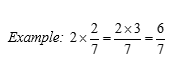While multiplying a whole number by a mixed fraction, change the mixed fraction into an improper fraction.To multiply two proper fractions, multiply their numerators and denominators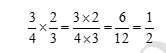Note:   When two proper fractions are multiplied, the product is less than each of the individual fractions. When two improper fractions are multiplied, the product is greater than each of the individual fractions.

Division of Fractions

To obtain the reciprocal of a fraction, interchange the numerator with the denominator.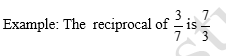To divide a whole number by a fraction, take the reciprocal of the fraction and then multiply it by the whole numberTo divide a fraction by a whole number, multiply the fraction by the reciprocal of the whole number.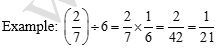To divide a fraction by a fraction, multiply the first fraction by the reciprocal of the second fraction.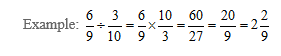To multiply a whole number by a decimal number, follow these steps:

1. Ignore the decimals and multiply the two numbers.
2. Count the numbers of digits to the right of decimal point in the original decimal number.
3. Insert the decimal, from right to left, in the answer by the same count.

Eg: (i)   3 × 0.2 = 0.6  (ii)    3 × 0.4 = 1.2

To multiply a decimal number by a decimal number, follow these steps:

1. Ignore the decimals and multiply the two numbers.
2. Count the number of digits to the right of decimal point in both the decimal numbers.
3. Add the number of digits counted and insert the decimal, from right to left, in the answer by the same count.

Eg: (i)   0.2 × 0.7 = 0.14  (ii)   0.9 × 0.02 = 0.018

To multiply a decimal number by 10, 100 or 1000, follow these steps:

1. While multiplying a decimal number by 10, retain the original number and shift the decimal to the right by one place.
1. While multiplying a decimal number by 100, retain the original number and shift the decimal to the right by two places.
1. While multiplying a decimal number by 1000, retain the original number and shift the decimal to the right by three places.

Division of Decimals

To divide a decimal number by a whole number, follow these steps:

1. Convert the decimal number into a fraction.
2. Take the reciprocal of the divisor.
3. Multiply the reciprocal by the fraction.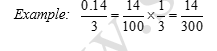To divide a decimal number by another decimal number, follow these steps:

1. Convert both the decimal numbers into fractions.
2. Take the reciprocal of the divisor.
3. Multiply the reciprocal by the fraction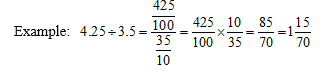## Tags:

Click for more Fractions and Decimals Study Material
 CBSE Class 7 Mathematics Fractions And Decimals Notes

## Latest NCERT & CBSE News

Read the latest news and announcements from NCERT and CBSE below. Important updates relating to your studies which will help you to keep yourself updated with latest happenings in school level education. Keep yourself updated with all latest news and also read articles from teachers which will help you to improve your studies, increase motivation level and promote faster learning

### CBSE Science Challenge 2021 22

Science is inexplicably linked with our lives and helps us to understand the world around us better. Scientific and technological developments contribute to progress and help improve our standards of living. By engaging with this subject, students learn to think, solve...

### Surya Namaskar Project on 75th Anniversary of Independence Day

Ministry of Education, Govt of India vide letter No. F.No. 12-5/2020-IS-4 dated 16.12.2021 has intimated that under the banner Azadi ka Amrit Mahotsav the National Yogasanasports Federation has decided to run a project of 750 million Surya Namaskar from 01 January 2022...

### National Youth Day and Birth Anniversary of Swami Vivekananda

Ministry of Education, Govt. of India vide D.O No. 12-4/2021-IS.4 dated 04.01.2022 intimated that 12 January 2022 will be celebrated as “National Youth Day” and “Birth Anniversary of Swami Vivekananda”.   All Schools affiliated to CBSE may celebrate 12 January 2022 as...

### Online courses for classes XI and XII offered by NCERT

Ministry of Education (MoE), Government of India has launched a platform for offering Massive Open Online Courses (MOOCs) that is popularly known as SWAYAM (Study Webs of Active learning for Young Aspiring Minds) on 9 th July, 2017. NCERT now offers online courses for...

### Celebration of Matribhasha Diwas Mother Language day

UNESCO has declared 21st February of every year to be celebrated as International Mother Language day to promote dissemination of Mother Language of all, create awareness of linguistic and cultural traditions and diversity across the world and to inspire solidarity...